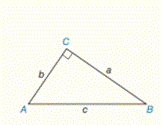Chapter 3.CT, Problem 7CT### Elementary Geometry for College St...

6th Edition
Daniel C. Alexander + 1 other
ISBN: 9781285195698

#### Solutions

Chapter
Section### Elementary Geometry for College St...

6th Edition
Daniel C. Alexander + 1 other
ISBN: 9781285195698
Textbook Problem
1 views

# In Δ A B C , m ∠ C = 90 ° Find :a) c if a = 8 and b = 6                                     _ b) b if a = 6 and c = 8 l                                     _

To determine

a)

To find:

The value of c.

Explanation

Procedure used:

In a right angle the sum of squares of two sides of a triangle is equal to the square of third longest side.

Given:

The given figure is,

Figure (1)

mC=90°, a=8

And,

b=6

Calculation:

Equate the sum of squares of two sides of the triangle is equal to the square of third longest side.

c2=a2+b2c=a

To determine

b)

To find:

The value of b.

### Still sussing out bartleby?

Check out a sample textbook solution.

See a sample solution

#### The Solution to Your Study Problems

Bartleby provides explanations to thousands of textbook problems written by our experts, many with advanced degrees!

Get Started

#### CHECK POINT 1. Complete the following. (a) (b) (c) (d) (e) (f)

Mathematical Applications for the Management, Life, and Social Sciences

#### True or False: If , then converge absolutely.

Study Guide for Stewart's Multivariable Calculus, 8th

#### True or False: is a convergent series.

Study Guide for Stewart's Single Variable Calculus: Early Transcendentals, 8th